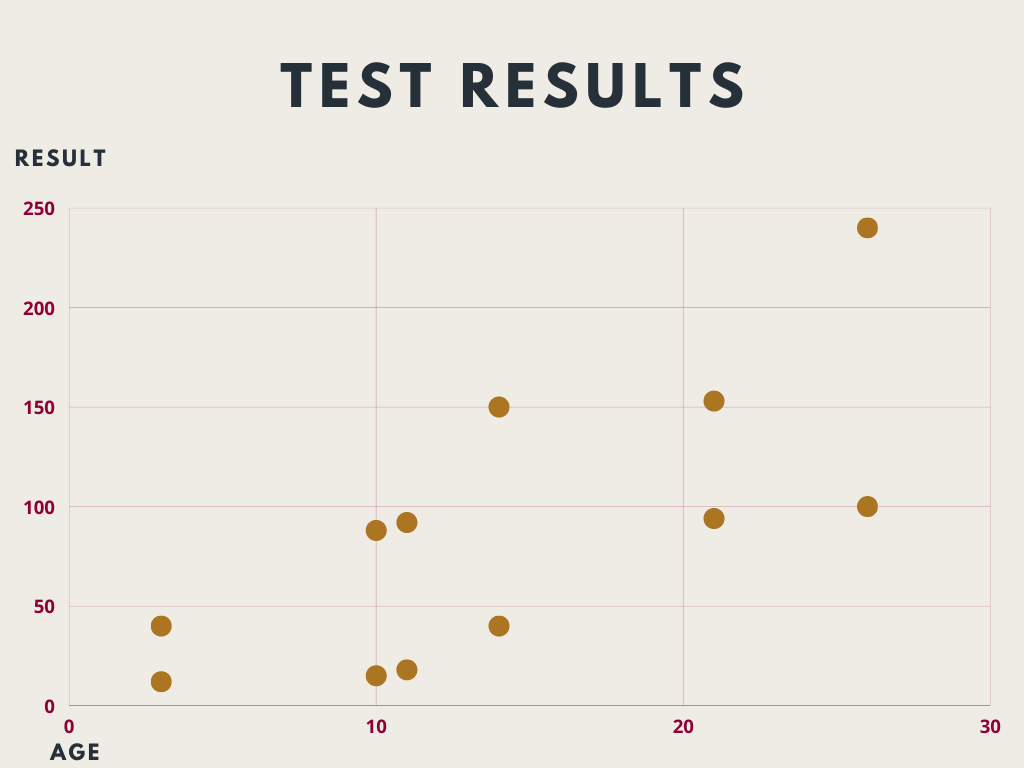Course Content

# First Dive into seaborn Visualization

First Dive into seaborn Visualization

##Scatterplot

A scatterplot uses dots to represent values for two different numeric variables. The position of each dot on the horizontal and vertical axis indicates values for an individual data point.

An example of a scatterplot:To initialize a scatterplot based on the `pandas` DataFrame, we need to input at least 3 parameters: `x`, `y` (columns-coordinates for the plot), and `data` (the DataFrame containing the data).

Look at the code below!Let's solve this problem!1. Import the `seaborn` with `sns` alias.
2. Import the `matplotlib.pyplot` with `plt` alias.
3. Import the `pandas` with `pd` alias.
4. Create a scatterplot using `'x'` column for the x-value and `'y'` column for the y-value.